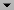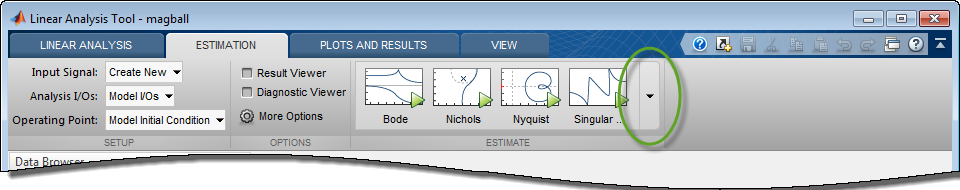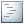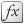## Generate MATLAB Code for Repeated or Batch Frequency Response Estimation

This topic shows how to generate MATLAB® code for frequency response estimation from the Model Linearizer. You can generate either a MATLAB script or a MATLAB function. Generated MATLAB scripts are useful when you want to programmatically reproduce a result you obtained interactively. A generated MATLAB function allows you to perform multiple estimations with systematic variations in estimation parameters such as operating point (batch estimation).

To generate MATLAB code for estimation:

1. In Model Linearizer, in the Estimation tab, interactively configure the input signal, analysis I/Os, operating point, and other parameters for frequency response estimation.

2. Clickto expand the gallery.3. Select the type of code you want to generate:

•`Script` — Generate a MATLAB script that uses your configured parameter values. Select this option when you want to repeat the same frequency response estimation at the MATLAB command line.

•`Function` — Generate a MATLAB function that takes analysis I/Os, operating points, and input signals as input arguments. Select this option when you want to perform multiple frequency response estimations using different parameter values (batch estimation).

To use a generated MATLAB function for batch estimation, you can create a MATLAB script with a `for` loop that cycles through values of the parameter you want to vary. Call the generated MATLAB function in each iteration of the loop.